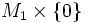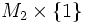# Framed cobordism of framed submanifolds

(diff) ← Older revision | Latest revision (diff) | Newer revision → (diff)
Suppose$M_1$ and$M_2$ are framed submanifolds of a differential manifold$P$. A framed cobordism between$M_1$ and$M_2$ in$P$ is a framed submanifold with boundary$(N, \partial N)$ inside$P \times I$, that provides a cobordism between$M_1 \times \{ 0 \}$ and$M_2 \times \{ 1 \}$.